# Stoichiometry Chemical Quantities Notes Stoichiometry Study of quantitative

• Slides: 16Stoichiometry – Chemical Quantities Notes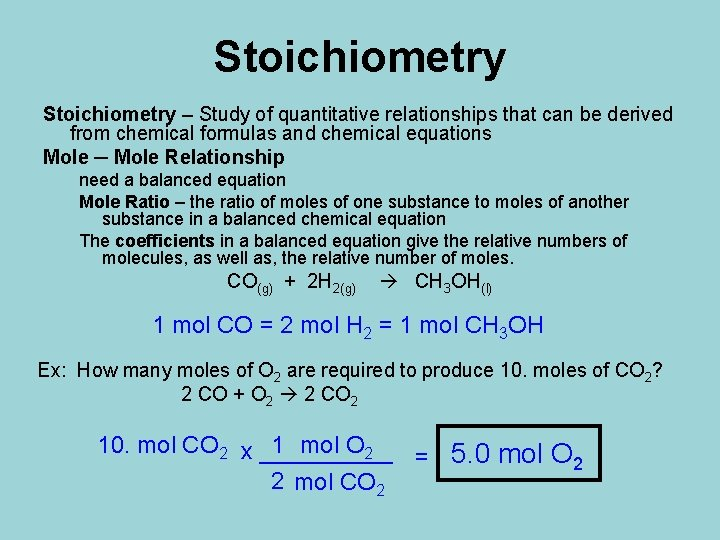Stoichiometry – Study of quantitative relationships that can be derived from chemical formulas and chemical equations Mole ─ Mole Relationship need a balanced equation Mole Ratio – the ratio of moles of one substance to moles of another substance in a balanced chemical equation The coefficients in a balanced equation give the relative numbers of molecules, as well as, the relative number of moles. CO(g) + 2 H 2(g) CH 3 OH(l) 1 mol CO = 2 mol H 2 = 1 mol CH 3 OH Ex: How many moles of O 2 are required to produce 10. moles of CO 2? 2 CO + O 2 2 CO 2 10. mol CO 2 x _____ 1 mol O 2 = 5. 0 mol O 2 2 mol CO 2What other relationships do we have for the mole? • 1 mol = 6. 02 x 1023 atoms / molecules / particles • 1 mol = [molar mass] g • 1 amu = 1. 66 x 10 -24 g We can add these mole relationships on either end of the mole ratio: # unit A x 1 mol A x mol B x __ unit B = # unit B _ unit A _ mol A 1 mol B mole relationship mole ratio (switch units) (switch substances) mole relationship (switch units) *A is the GIVEN substance & B is the WANTED substance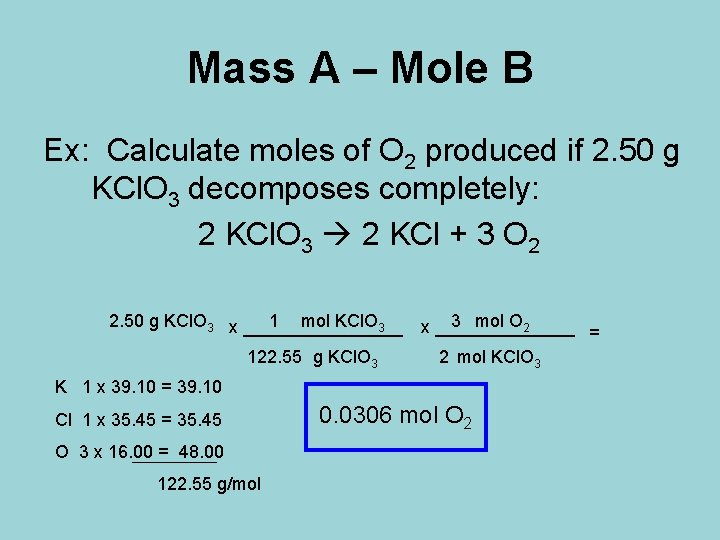Mass A – Mole B Ex: Calculate moles of O 2 produced if 2. 50 g KCl. O 3 decomposes completely: 2 KCl. O 3 2 KCl + 3 O 2 2. 50 g KCl. O 3 x ________ 1 mol KCl. O 3 3 mol O 2 x _______ = 122. 55 g KCl. O 3 2 mol KCl. O 3 K 1 x 39. 10 = 39. 10 Cl 1 x 35. 45 = 35. 45 O 3 x 16. 00 = 48. 00 122. 55 g/mol 0. 0306 mol O 2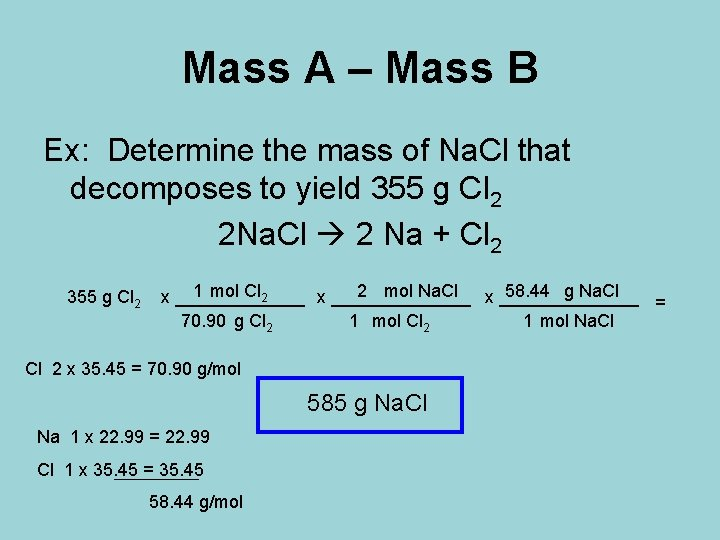Mass A – Mass B Ex: Determine the mass of Na. Cl that decomposes to yield 355 g Cl 2 2 Na. Cl 2 Na + Cl 2 355 g Cl 2 1 mol Cl 2 2 mol Na. Cl 58. 44 g Na. Cl x ______________ x _______ = 70. 90 g Cl 2 1 mol Na. Cl Cl 2 x 35. 45 = 70. 90 g/mol 585 g Na. Cl Na 1 x 22. 99 = 22. 99 Cl 1 x 35. 45 = 35. 45 58. 44 g/molMole A – Mass B Ex: Calculate the number of grams of oxygen required to react exactly with 4. 30 mol of propane, C 3 H 8, in the reaction by the following balanced equation: C 3 H 8(g) + 5 O 2(g) 3 CO 2(g) + 4 H 2 O(g) 32. 00 g O 2 5 mol O 2 4. 30 mol C 3 H 8 x _______ x _____ 1 mol C 3 H 8 1 mol O 2 x 16. 00 = 32. 00 g/mol 688 g O 2 =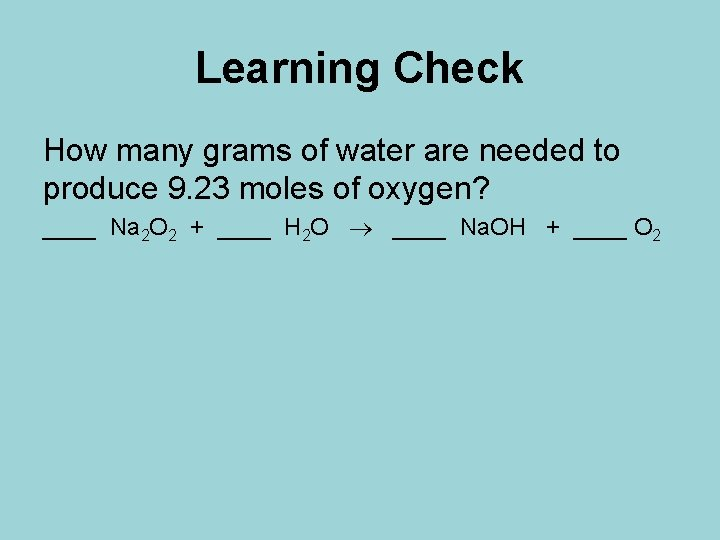Learning Check How many grams of water are needed to produce 9. 23 moles of oxygen? ____ Na 2 O 2 + ____ H 2 O ____ Na. OH + ____ O 22. Limiting Reactant & Percent YieldBackground Knowledge Check Label the reactant(s) and product(s) in the following reaction: 2 Mg + O 2 2 Mg. O Reactant(s): Mg and O 2 Product(s): Mg. O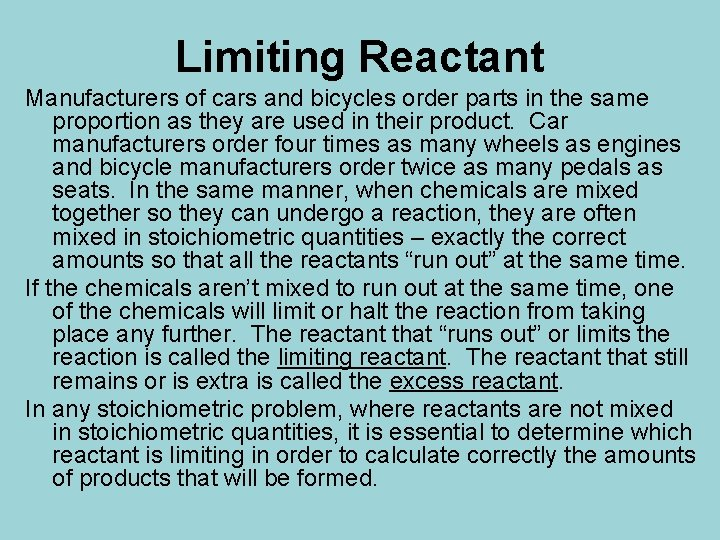Limiting Reactant Manufacturers of cars and bicycles order parts in the same proportion as they are used in their product. Car manufacturers order four times as many wheels as engines and bicycle manufacturers order twice as many pedals as seats. In the same manner, when chemicals are mixed together so they can undergo a reaction, they are often mixed in stoichiometric quantities – exactly the correct amounts so that all the reactants “run out” at the same time. If the chemicals aren’t mixed to run out at the same time, one of the chemicals will limit or halt the reaction from taking place any further. The reactant that “runs out” or limits the reaction is called the limiting reactant. The reactant that still remains or is extra is called the excess reactant. In any stoichiometric problem, where reactants are not mixed in stoichiometric quantities, it is essential to determine which reactant is limiting in order to calculate correctly the amounts of products that will be formed.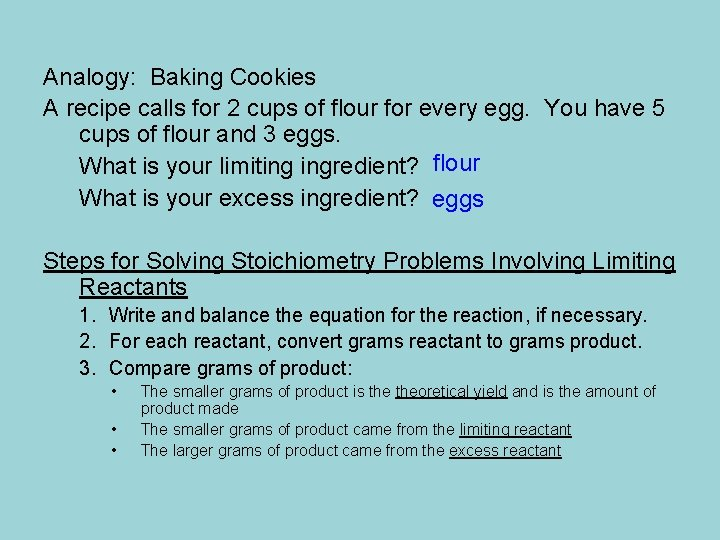Analogy: Baking Cookies A recipe calls for 2 cups of flour for every egg. You have 5 cups of flour and 3 eggs. What is your limiting ingredient? flour What is your excess ingredient? eggs Steps for Solving Stoichiometry Problems Involving Limiting Reactants 1. Write and balance the equation for the reaction, if necessary. 2. For each reactant, convert grams reactant to grams product. 3. Compare grams of product: • • • The smaller grams of product is theoretical yield and is the amount of product made The smaller grams of product came from the limiting reactant The larger grams of product came from the excess reactant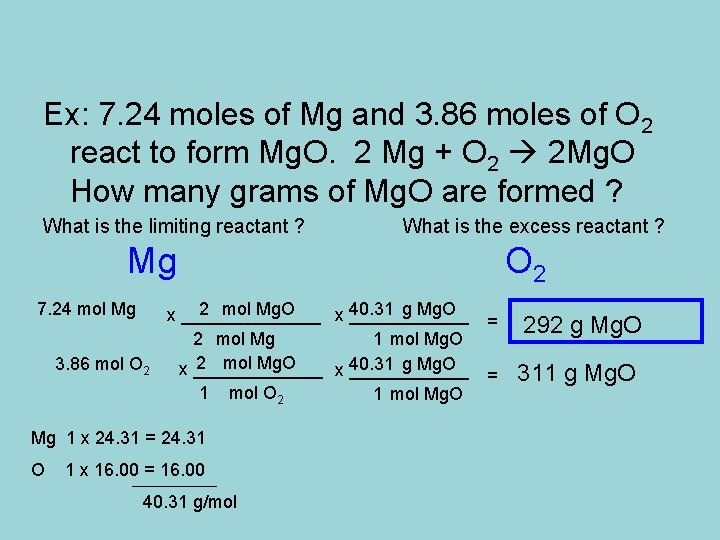Ex: 7. 24 moles of Mg and 3. 86 moles of O 2 react to form Mg. O. 2 Mg + O 2 2 Mg. O How many grams of Mg. O are formed ? What is the limiting reactant ? What is the excess reactant ? Mg 7. 24 mol Mg 3. 86 mol O 2 2 mol Mg. O 40. 31 g Mg. O x _______ x ______ = 2 mol Mg 1 mol Mg. O 2 mol Mg. O 40. 31 g Mg. O x _______ x ______ = 1 mol O 2 1 mol Mg. O Mg 1 x 24. 31 = 24. 31 O 1 x 16. 00 = 16. 00 40. 31 g/mol O 2 292 g Mg. O 311 g Mg. OEx: Suppose 2. 50 x 104 g of N 2 and 5. 00 x 103 g of H 2 are mixed and reacted to form ammonia. Calculate the mass of ammonia produced when the reaction is run to completion. N 2 + 3 H 2 2 NH 3 What is the limiting reactant? What is the excess reactant? H 2 N 2 2. 50 x 104 g N 2 x ______ 1 mol N 2 x ______ 17. 04 g NH 3 2 mol NH 3 x _______ 28. 02 g N 2 1 mol NH 3 = 3. 04 x 104 g NH 3 17. 04 g NH 3 5. 00 x 103 g H 2 x ______ 1 mol H 2 x ______ 2 mol NH 3 x _______ 2. 02 g H 2 3 mol H 2 1 mol NH 3 = N 2 x 14. 01 = 28. 02 g/mol H 2 x 1. 01 = 2. 02 g/mol N 1 x 14. 01 = 14. 01 H 3 x 1. 01 = 3. 03 17. 04 g/mol 2. 81 x 104 g NH 3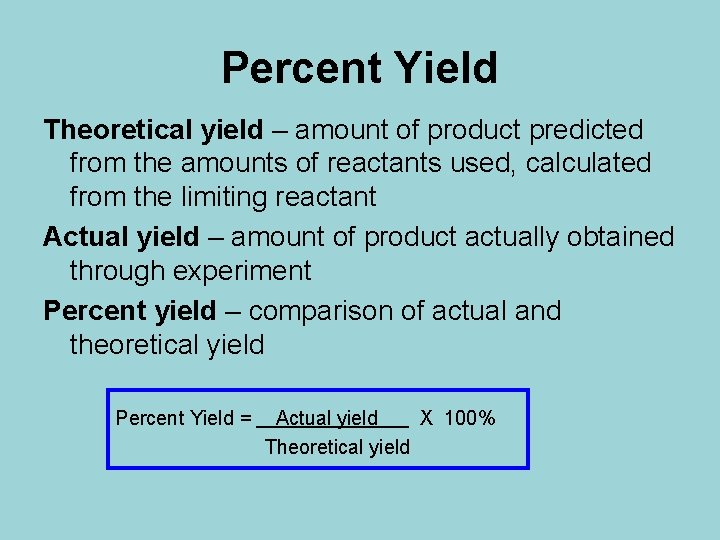Percent Yield Theoretical yield – amount of product predicted from the amounts of reactants used, calculated from the limiting reactant Actual yield – amount of product actually obtained through experiment Percent yield – comparison of actual and theoretical yield Percent Yield = Actual yield X 100% Theoretical yield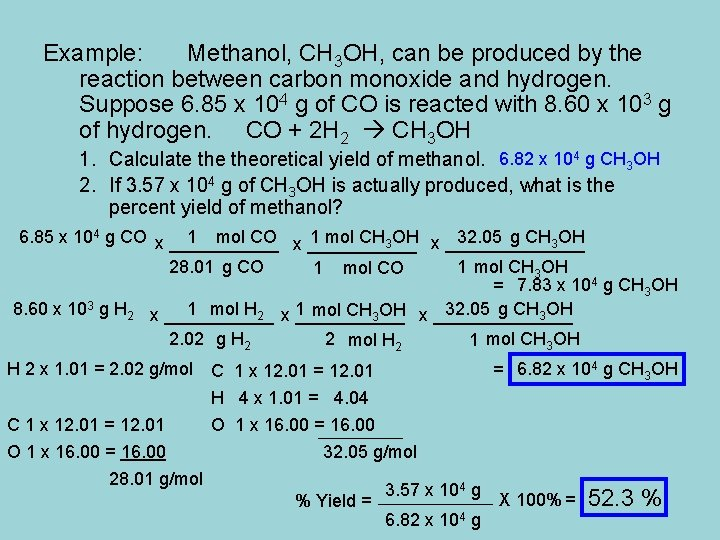Example: Methanol, CH 3 OH, can be produced by the reaction between carbon monoxide and hydrogen. Suppose 6. 85 x 104 g of CO is reacted with 8. 60 x 103 g of hydrogen. CO + 2 H 2 CH 3 OH 1. Calculate theoretical yield of methanol. 6. 82 x 104 g CH 3 OH 2. If 3. 57 x 104 g of CH 3 OH is actually produced, what is the percent yield of methanol? 6. 85 x 104 g CO x ___________ 1 mol CH 3 OH x _______ 32. 05 g CH 3 OH 28. 01 g CO 1 mol CH 3 OH 1 mol CO = 7. 83 x 104 g CH 3 OH 8. 60 x 103 g H 2 x ___________ 1 mol CH 3 OH x _______ 32. 05 g CH 3 OH 2. 02 g H 2 x 1. 01 = 2. 02 g/mol C 1 x 12. 01 = 12. 01 O 1 x 16. 00 = 16. 00 28. 01 g/mol 2 mol H 2 1 mol CH 3 OH C 1 x 12. 01 = 12. 01 H 4 x 1. 01 = 4. 04 O 1 x 16. 00 = 16. 00 32. 05 g/mol % Yield = 3. 57 x 104 g 6. 82 x 104 g = 6. 82 x 104 g CH 3 OH X 100% = 52. 3 %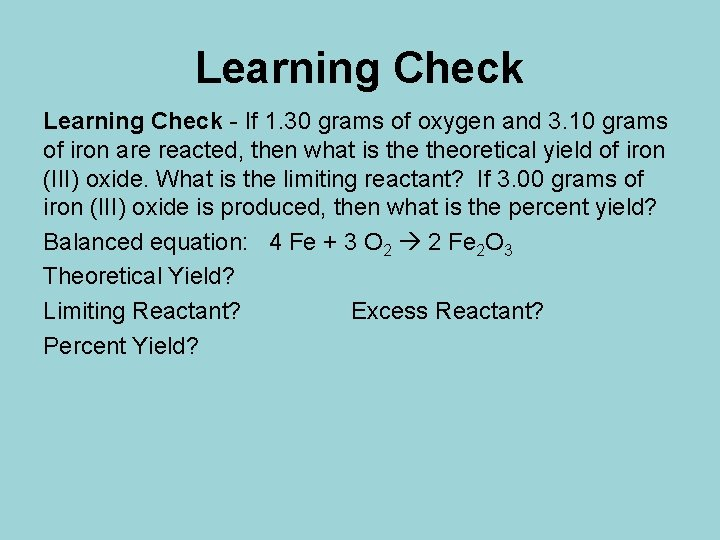Learning Check - If 1. 30 grams of oxygen and 3. 10 grams of iron are reacted, then what is theoretical yield of iron (III) oxide. What is the limiting reactant? If 3. 00 grams of iron (III) oxide is produced, then what is the percent yield? Balanced equation: 4 Fe + 3 O 2 2 Fe 2 O 3 Theoretical Yield? Limiting Reactant? Excess Reactant? Percent Yield?